TitleCollege Algebra
FunctionsLearning Objectives

 After completing this tutorial, you should be able to: Find the vertex of a quadratic function. Determine if the vertex is the maximum or minimum point of a quadratic function. Graph a quadratic function.Introduction

 In this tutorial we will be looking at graphs of quadratic functions.  The graph of a quadratic function is called a parabola and has a curved shape.  One of the main points of a parabola is its vertex.  It is the highest or the lowest point on its graph.  You can think of like an endpoint of a parabola.  I will be showing you how to find the vertex as well as the axis of symmetry that goes through this point.  I will also refresh your memory on how to find the x- and y- intercepts.  If you need a review on what intercepts on a graph are, feel free to go to Tutorial 26: Equations of Lines.  When finding x-intercepts, you will find yourself having to solve a quadratic equation.  If you need a review on solving quadratic equations, feel free to go to Tutorial 17: Quadratic Equations.  If you are in a college algebra class, working with quadratic functions is inevitable, even if it is against your religion.  So I guess you better get started.Tutorial

 Quadratic Function A quadratic function is a function that  can be written in the form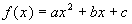where a, b, and c are constants and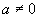Note that in a quadratic function there is a power of two on your independent variable and that is the highest power.

 Standard Form of a  Quadratic Function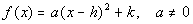Sometimes your quadratic function is written in standard form.  It is ok to leave it in this form when working with your problem.  I will be showing you how to graph a parabola using either form.

 The graph of a quadratic function is called a parabola.  It is basically a curved shape opening up or down.

 What does a tell us?

 When you have a quadratic function in either form,OR, if a > 0, then the parabola opens up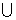, if a < 0, then the parabola opens down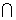.

 Vertex

The vertex is the lowest or highest point (depending on direction) on the graph of a quadratic function.

Finding the vertex using the form,:

 If your quadratic function is in the form,,  then the      vertex =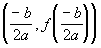.  Basically you will find the x value of the vertex first and then just plug that value into the function to get the y or functional value of the vertex.

Finding the vertex using the form:

 If your quadratic function is in the form, then the  vertex = (h, k).

 Axis of Symmetry

 Each parabola is symmetric about a vertical line called the axis of symmetry.  This vertical line goes through the vertex. Think of it as a mirrored image about this vertical line.

 The next three graphs illustrate the different aspects of the graph of a quadratic function or parabola.

The following is the graph of the function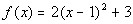: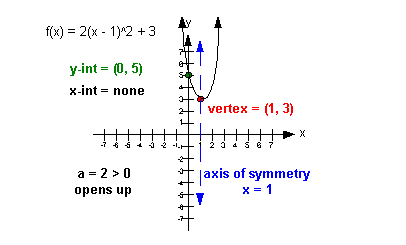First of all, see how the vertex is the lowest point on the graph.  It is either going to be the lowest or highest point on the graph of a quadratic function. Second, look at the axis of symmetry.  It is not actually part of the graph itself, but is important in that the parabola creates a mirrored image about it.  Note how it is symmetric about the axis of symmetry.  Also, note how it goes through the vertex.  Third, note how there is one y-intercept but no x-intercept.  The quadratic function can have no, one or two x-intercepts.

The following is the graph of the function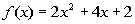:First of all, see how the vertex is the lowest point on the graph.  It is either going to be the lowest or highest point on the graph of a quadratic function. Second, look at the axis of symmetry.  It is not actually part of the graph itself, but is important in that the parabola creates a mirrored image about it.  Note how it is symmetric about the axis of symmetry.  Also, note how it goes through the vertex.  Third, note how there is one y-intercept and one x-intercept.  The quadratic function can have no, one or two x-intercepts.

The following is the graph of the function: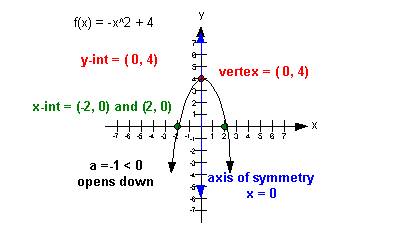First of all, see how the vertex is the highest point on the graph.  It is either going to be the lowest or highest point on the graph of a quadratic function. Second, look at the axis of symmetry.  It is not actually part of the graph itself, but is important in that the parabola creates a mirrored image about it.  Note how it is symmetric about the axis of symmetry.  Also, note how it goes through the vertex.  Third, note how there is one y-intercept and two x-intercepts.  The quadratic function can have no, one or two x-intercepts.Example 1: Find the coordinates of the vertex of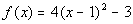.  Without graphing, determine if the vertex is the maximum or minimum point of the quadratic function.

 Vertex Note how this quadratic function is written in standard form.  That means we can find the vertex by lining it up with the general standard form and identify (h, k).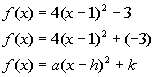*Standard form of quad. function

 Since (h, k) is the vertex in standard form, what do you think our vertex is for this problem? If you said (1, -3) you are correct.    Be careful about your signs on this problem.  It is real tempting to say that the vertex is (1, 3).  However take a close look at the standard form.  Notice how the sign in front of h is a minus, but the one in front of k is positive.  So h is the number we are subtracting from x, which in our case is 1.  k is the number we are adding at the end, which our case we are adding a negative 3.

 Maximum or Minimum? Next we want to determine if the vertex that we found, (1, -3), is a maximum or minimum point, without graphing. If we know which direction the curve opens, that can help us answer this question.  Since a = 4, and 4 is greater than 0, this parabola would open up. So does that mean the vertex is a maximum or minimum point? If you said a minimum point, you are right on.   So our vertex (1, -3) is the minimum point.Example 2: Find the coordinates of the vertex of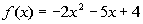.  Without graphing, determine if the vertex is the maximum or minimum point of the quadratic function.

 Vertex Note how this quadratic function is written in the form.  That means we can find the vertex by using the formula.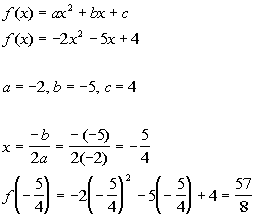*Identify a, b, and c   *Plug values into vertex form. for a, b, and c   *Plug -5/4 in for x to find the y value of the vertex

 The vertex would be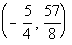.

 Maximum or Minimum? Next we want to determine if the vertex that we found,, is a maximum or minimum point, without graphing. If we know which direction the curve opens, that can help us answer this question.  Since a = -2, and -2 is less than 0, this parabola would open down. So does that mean the vertex is a maximum or minimum point? If you said a maximum point, you are right on.   So our vertexis the maximum point.

 Step 2: Find the vertex.

 Step 3: Find the intercepts.

 If you need a review on intercepts feel free to go to Tutorial 26: Equations of Lines.

 Step 4: Graph the parabola.

 Plot the points found in steps 2 and 3 and draw a curved line through them.Example 3: Use the vertex and the intercepts to sketch the graph of the quadratic function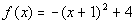.  Find the equation for this function's axis of symmetry.

 Since a = -1 and -1 < 0, then it looks like it is going to curve down. This gives us a good reference to know we are going in the right direction.

 Step 2: Find the vertex.

 Just like in example 1 above,  this quadratic function is written in standard form.  That means we can find the vertex by lining it up with the general standard form and identify (h, k).*Standard form of quad. function

 Since (h, k) is the vertex in standard form, what do you think our vertex is? If you said (-1, 4) you are correct.  Be careful about your signs on this problem.   Notice how the sign in front of h is a minus, but the one in front of k is positive.  So h is the number we are subtracting from x, which in our case is -1.  k is the number we are adding at the end, which our case we are adding a 4.

 Step 3: Find the intercepts.

 y-intercept Reminder that the y-intercept is always where the graph crosses the y-axis which means x = 0: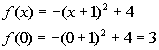*Replace x with 0

 The y-intercept is (0, 3).   x-intercept Reminder that the x-intercept is always where the graph crosses the x-axis which means y = 0: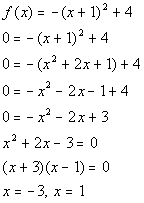*Replace y (or f(x)) with 0

 The x-intercepts are (-3, 0) and (1, 0).

 Step 4: Graph the parabola.Axis of symmetry As shown on the graph, the axis of symmetry is x = -1.Example 4: Use the vertex and the intercepts to sketch the graph of the quadratic function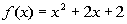.  Find the equation for this function's axis of symmetry.

 Since a = 1 and 1 > 0, then it looks like it is going to curve up. This gives us a good reference to know we are going in the right direction.

 Step 2: Find the vertex.

 Just like in example 2 above,  this quadratic function is written in the form.  That means we can find the vertex by using the formula.*Identify a, b, and c   *Plug values into vertex form. for a, b, and c   *Plug -1 in for x to find the y value of the vertex

 So the vertex is (-1, 1).

 Step 3: Find the intercepts.

 y-intercept Reminder that the y-intercept is always where the graph crosses the y-axis which means x = 0:*Replace x with 0

 The y-intercept is (0, 2).   x-intercept Reminder that the x-intercept is always where the graph crosses the x-axis which means y = 0: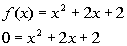*Replace y (or f(x)) with 0

 Note that this does not factor.  Let's try solving by using the quadratic formula: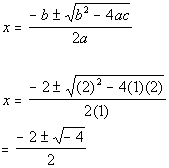*Plug in values for a, b, and c

 Note how we got a negative number underneath the square root.  That means there is no real number solution.  That also means that there are NO x-intercepts.

 Step 4: Graph the parabola.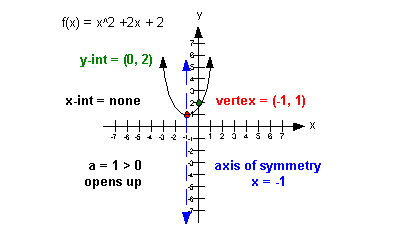Axis of symmetry As shown on the graph, the axis of symmetry is x = -1.Practice Problems

 These are practice problems to help bring you to the next level.  It will allow you to check and see if you have an understanding of these types of problems. Math works just like anything else, if you want to get good at it, then you need to practice it.  Even the best athletes and musicians had help along the way and lots of practice, practice, practice, to get good at their sport or instrument.  In fact there is no such thing as too much practice. To get the most out of these, you should work the problem out on your own and then check your answer by clicking on the link for the answer/discussion for that  problem.  At the link you will find the answer as well as any steps that went into finding that answer.Practice Problems 1a - 1b: Find the coordinates of the vertex of the given quadratic function.  Without graphing, determine if the vertex is the maximum or minimum point of the quadratic function.

 1a.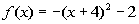(answer/discussion to 1a) 1b.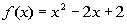(answer/discussion to 1b)Practice Problems 2a - 2b: Use the vertex and the intercepts to sketch the graph of the given quadratic function.  Find the equation for this function's axis of symmetry.

 2a.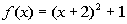(answer/discussion to 2a) 2b.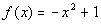(answer/discussion to 2b)Need Extra Help on these Topics?

The following is a webpage that can assist you in the topics that were covered on this page:

 http://www.purplemath.com/modules/grphquad.htm This webpage helps you with graphing parabolas.

Last revised on July 10, 2010 by Kim Seward.